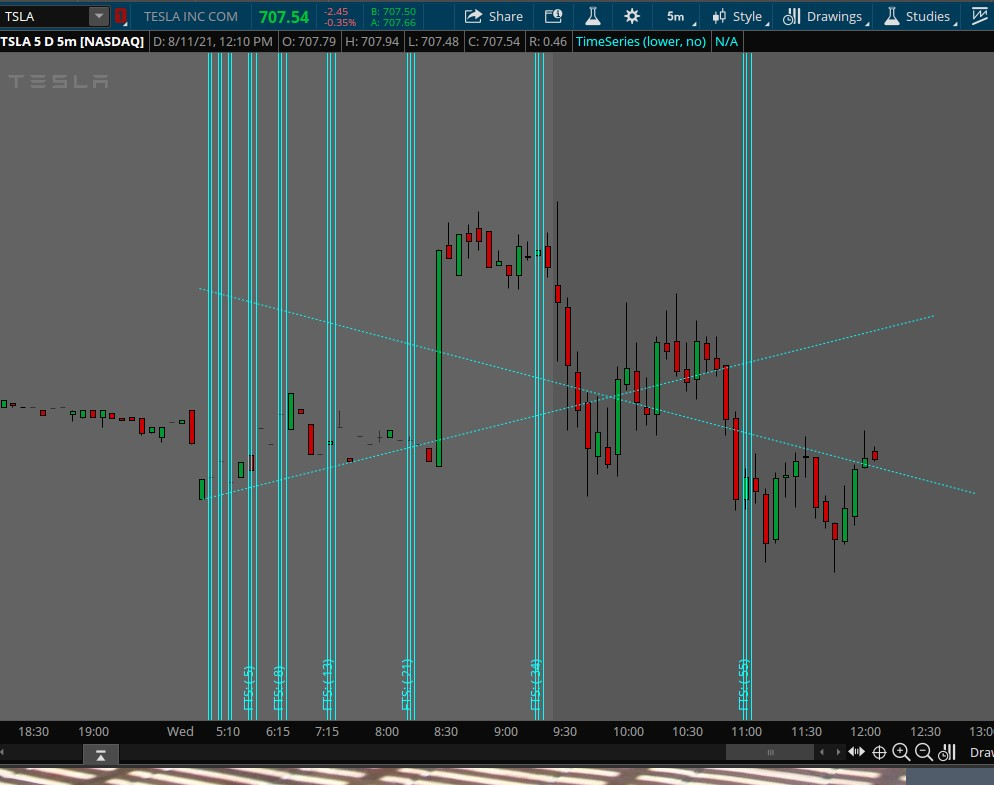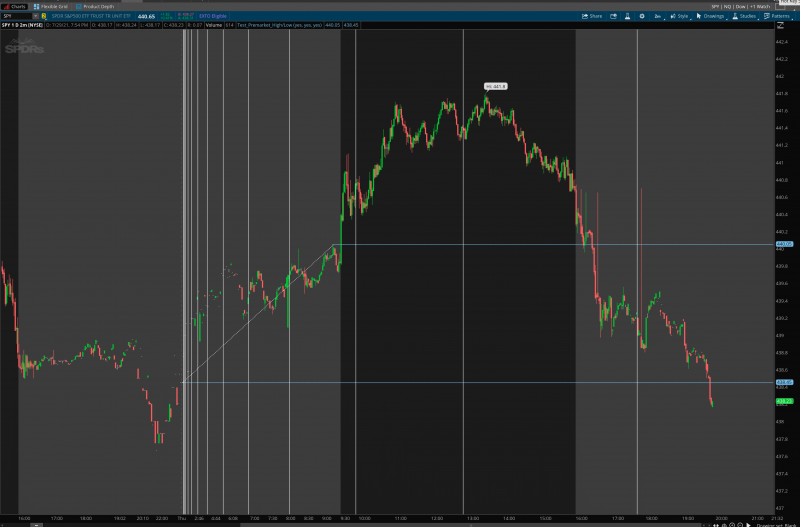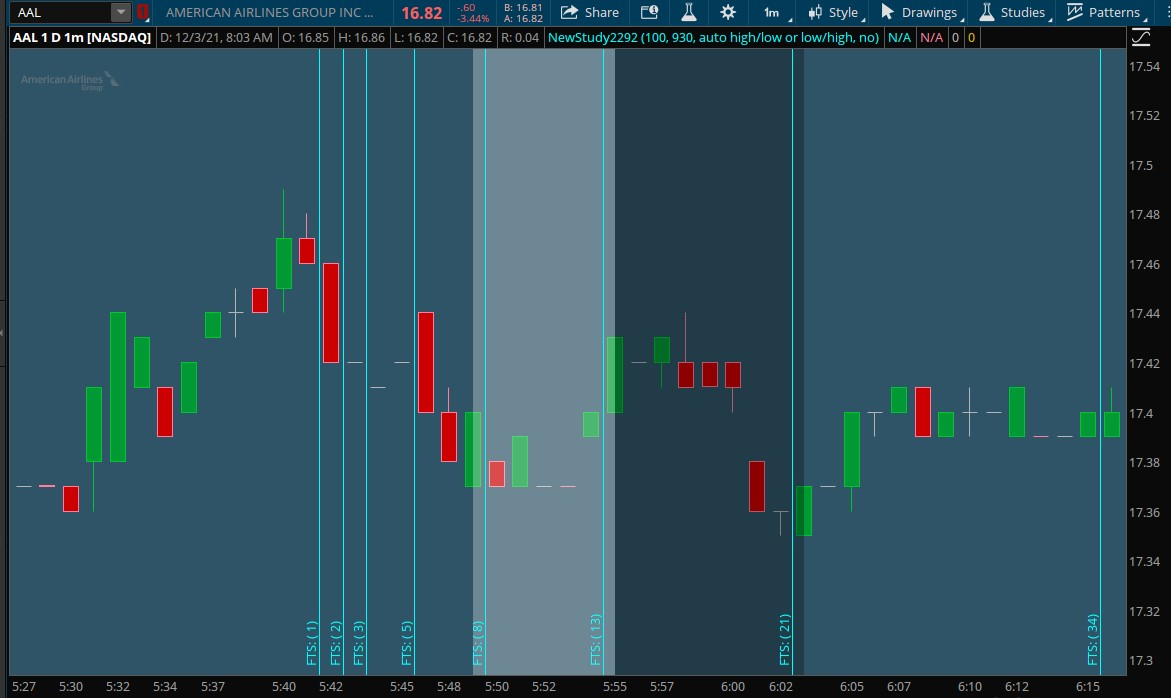# Mobius Fibonacci Time Series For ThinkOrSwim

Auto Fibonacci Time Series
A few years ago, Messy Jesse, a TOS employee appeared on the Mr. Script show at that time and provided as part of his fibonacci retracement code to which Mobius added some fibonacci timeseries code. http://tos.mx/zePls0G

I have hopefully modifed the timeseries portion to include your idea. The modification will find the higher or lower barnumber occurrence of the premarket high or low and use that as the starting point for plotting the timeseries.

The only comparison I used was the TOS timeseries drawing tool. It seems to draw a starting point where the lower barnumber of the high or low is clicked, which might mean to use the lower barnumber occurrence of the premarket high or low. I have included an option so you can choose either higher or lower as a starting point, with the lower defaulted.

In the chart below is the vertical lines created by the code below and 2 different timeseries drawn from left to right or right to left with the lower premarket chosen and all appear to provide similar resultsRuby:
``````# Time Series provided by Mobius
# Modified to start with the higher barnumber associated with premarket high or premarket low

def barnumber = BarNumber();
def premarket = SecondsFromTime(0400) >= 0 and SecondsTillTime(0930) > 0;
def prehigh   = if premarket == 0 and premarket then high else if premarket then  Max(high, prehigh) else 0;
def prelow    = if premarket == 0 and premarket then low else if premarket then Min(low, prelow) else 0;
def highnumber = if high == prehigh then BarNumber() else Double.NaN;
def lownumber  = if low == prelow   then BarNumber() else Double.NaN;

# Fibonacci Time Series
input starting_point = {default lower, higher};
def start = if starting_point == starting_point.higher then Max(HighestAll(highnumber), HighestAll(lownumber)) else Min(HighestAll(highnumber), HighestAll(lownumber));
rec bar1 = if start == BarNumber()
then BarNumber()
else bar1;
rec bars = if BarNumber() == bar1
then 2
else if barnumber > bar1
then bars + 1
else 0;

def coeff = Sqrt(5);
def smallest = 5;
def n = Floor(Log(bars * coeff + 0.5) / Log((1 + Sqrt(5)) / 2));
def inSeries = n != n and bars >= smallest;
def Series = if inSeries
then bars
else Double.NaN;
AddVerticalLine(GetDay() == GetLastDay() and Series, "FTS: ( " + Series + ")", Color.CYAN, Curve.FIRM);

input debug = no;
AddLabel(debug, HighestAll(highnumber) + " " + HighestAll(lownumber) + " " + bar1, Color.WHITE);
plot x = if debug then barnumber else Double.NaN;
x.SetPaintingStrategy(PaintingStrategy.VALUES_BELOW);
#``````

Last edited by a moderator:
•SJP07 and MerryDay

#### SJP07

##### Member
I'm not a coder, but I would appreciate it if someone can create a code for me using the Fibonacci time series drawing. I would like to have the begin and end point automatically connected to the pre-market high and low. Thanks in advance!Last edited by a moderator:
Thank you so much!!!!!!! I'm so thankful for you. Is there anyway to have it automatically switch from low to high or high to low? Something similar to this: https://usethinkscript.com/threads/plot-range-of-first-15min.2657/

Thanks again!

You're welcome.

It does automatically switch between high and low, it is just whether you want it to choose the last one that occurs, then choose higher. If you want whatever occurred first between high and low, then choose lower.

Using the timeseries drawing tool seems in my testing to always plot whatever is to the left no matter whether you start from the left or start from the right and go left. So the default is 'lower' using whatever occurred first, either the low or high, which seemed to match the drawing tool irrespective of how you would draw it.

However, If you want the timeseries to start with whatever occurred last, either the high or low then choose 'higher'

The distance between the high and low seems to have no bearing on the timeseries.

If you have some other method, please describe it in detail.

Thank you so much!!!!!!! I'm so thankful for you. Is there anyway to have it automatically switch from low to high or high to low? Something similar to this: https://usethinkscript.com/threads/plot-range-of-first-15min.2657/

Thanks again!

I think you want an option that I have not seen the drawing tool do, but I think this will work. It will automatically if there is a high to low, it will choose the start at the low and if low to high, it will choose the start at high. I changed lower and higher to first and last to better match what they do.

See if this works how you expected it to work

Ruby:
``````# Time Series provided by Mobius
# Modified to start with the higher barnumber associated with premarket high or premarket low

def barnumber = BarNumber();
def premarket = SecondsFromTime(0400) >= 0 and SecondsTillTime(0930) > 0;
def prehigh   = if premarket == 0 and premarket then high else if premarket then  Max(high, prehigh) else 0;
def prelow    = if premarket == 0 and premarket then low else if premarket then Min(low, prelow) else 0;
def highnumber = HighestAll(if high == prehigh then BarNumber() else Double.NaN);
def lownumber  = HighestAll(if low == prelow   then BarNumber() else Double.NaN);

# Fibonacci Time Series
input starting_point = {default first, last, "auto high/low or low/high"};
def start;
switch (starting_point){
case first:
start = Min(highnumber, lownumber);
case last:
start = Max(highnumber, lownumber);
case "auto high/low or low/high":
start = if highnumber < lownumber then lownumber else highnumber ;
}
rec bar1 = if start == BarNumber()
then BarNumber()
else bar1;
rec bars = if BarNumber() == bar1
then 2
else if barnumber > bar1
then bars + 1
else 0;

def coeff = Sqrt(5);
def smallest = 5;
def n = Floor(Log(bars * coeff + 0.5) / Log((1 + Sqrt(5)) / 2));
def inSeries = n != n and bars >= smallest;
def Series = if inSeries
then bars
else Double.NaN;
AddVerticalLine(GetDay() == GetLastDay() and Series, "FTS: ( " + Series + ")", Color.CYAN, Curve.FIRM);

input debug = no;
AddLabel(debug, HighestAll(highnumber) + " " + HighestAll(lownumber) + " " + bar1, Color.WHITE);
plot x = if debug then barnumber else Double.NaN;
x.SetPaintingStrategy(PaintingStrategy.VALUES_BELOW);
#``````

•SJP07
I changed the premarket to 1700 and 0100.
now it's not working any advice?

I changed the premarket to 1700 and 0100.
now it's not working any advice?

The code was written to a specific request and wrapping time around midnight is a little tricky. Here is a revision using Linus' wrapping midnight code to hopefully create a variable opening/closing time scenario.

Ruby:
``````# Time Series provided by Mobius
# Modified to start with the higher barnumber associated with inrange high or inrange low

def barnumber = BarNumber();
input openingTime = 1700;
input closingTime = 0100;

#Linus logic to wrap time around midnight to allow variable user inputs for time
def sec1 = secondsFromTime(openingTime);
def sec2 = secondsFromTime(closingTime);
def isTime1 = (sec1 >= 0 and sec1 < 0) or (sec1 < sec1 and sec1 >= 0);
def isTime2 = (sec2 >= 0 and sec2 < 0) or (sec2 < sec2 and sec2 >= 0);
def inRange = compoundValue(1, if isTime1 then 1 else if isTime2 then 0 else inRange, 0);

def rangehigh   = if inrange == 0 and inrange then high else if inrange then  Max(high, rangehigh) else 0;
def rangelow    = if inrange == 0 and inrange then low else if inrange then Min(low, rangelow) else 0;
def highnumber  = HighestAll(if high == rangehigh then BarNumber() else Double.NaN);
def lownumber   = HighestAll(if low == rangelow   then BarNumber() else Double.NaN);

# Fibonacci Time Series
input starting_point = {default first, last, "auto high/low or low/high"};
def start;
switch (starting_point){
case first:
start = Min(highnumber, lownumber);
case last:
start = Max(highnumber, lownumber);
case "auto high/low or low/high":
start = if highnumber < lownumber then lownumber else highnumber ;
}
rec bar1 = if start == BarNumber()
then BarNumber()
else bar1;
rec bars = if BarNumber() == bar1
then 2
else if barnumber > bar1
then bars + 1
else 0;

def coeff = Sqrt(5);
def smallest = 5;
def n = Floor(Log(bars * coeff + 0.5) / Log((1 + Sqrt(5)) / 2));
def inSeries = n != n and bars >= smallest;
def Series = if inSeries
then bars
else Double.NaN;
AddVerticalLine(GetDay() == GetLastDay() and Series, "FTS: ( " + Series + ")", Color.CYAN, Curve.FIRM);

input debug = no;
AddLabel(debug, HighestAll(highnumber) + " " + HighestAll(lownumber) + " " + bar1, Color.WHITE);
plot x = if debug then barnumber else Double.NaN;
x.SetPaintingStrategy(PaintingStrategy.VALUES_BELOW);
plot y = if debug then inrange else double.nan;
y.setpaintingStrategy(paintingStrategy.VALUES_ABOVE);
#``````

Last edited:
•TonXas
Hi guys...for day & swing trading purposes, what this ideal time frame to use for this tool?

Hi guys...for day & swing trading purposes, what this ideal time frame to use for this tool?

I don't swing trade (never know what could happen overnight) but I find this tool the most useful on the 5min time frame.

•SleepyZ
Hi again!

This has been working so well. Is there a way to hide some of the lines so that the 34, 55, 80, etc are the only ones that show? Thanks in advance!

Last edited by a moderator:
Hi again!

This has been working so well. Is there a way to hide some of the lines so that the 34, 55, 80, etc are the only ones that show? Thanks in advance!

Replace the following line which has the change: Series>=34. Glad it sees to be working for you!

Ruby:
``AddVerticalLine(GetDay() == GetLastDay() and Series>=34, "FTS: ( " + Series + ")", Color.CYAN, Curve.FIRM);``

@SleepyZ Is there a reason why some of the vertical lines repeat pre-market?

Last edited by a moderator:
@SleepyZ Is there a reason why some of the vertical lines repeat pre-market?

I would need to see what symbol and chart settings you have seen this happen.

I just ran all of my watchlist symbols in ONDEMAND with this script on a 30day, 5min chart on a prior days data and could not duplicate what you are seeing.

I can't paste an image for some reason, but here's a link of the screenshot I tried to upload: https://we.tl/t-XWXHJk0wLJ

I do not know what timeframe you were using, but it looks like 1d1m nor do I know what option you choose to plot the series. Since it is the weekend and TOS is in maintenance mode, it is hard to test or explain anything. It appears that for NIO, it is plotting both the correct series from the lownumber, but also the highnumber. I tried to get it to plot just the lownumber basis by changing all the start definitions to lownumber, but because the 'n' (this is the guru Mobius' calc) changed at 0930 (possible bad TOS data), it still plots both on NIO. I tested all of my watchlist stocks and found no problems otherwise.

If you are still having unexplained stuff during a trading day, especially with NIO, share your grid and post it here, so I can replicate the exact situation.

I do not know what timeframe you were using, but it looks like 1d1m nor do I know what option you choose to plot the series. Since it is the weekend and TOS is in maintenance mode, it is hard to test or explain anything. It appears that for NIO, it is plotting both the correct series from the lownumber, but also the highnumber. I tried to get it to plot just the lownumber basis by changing all the start definitions to lownumber, but because the 'n' (this is the guru Mobius' calc) changed at 0930 (possible bad TOS data), it still plots both on NIO. I tested all of my watchlist stocks and found no problems otherwise.

If you are still having unexplained stuff during a trading day, especially with NIO, share your grid and post it here, so I can replicate the exact situation.
Hi again! Is there a way to add clouds in between series? For example, I want a white cloud between series 8 and 13 and a black cloud between 13 and 21.

Hi again! Is there a way to add clouds in between series? For example, I want a white cloud between series 8 and 13 and a black cloud between 13 and 21.

This might helpRuby:
``````# Time Series provided by Mobius
# Modified to start with the higher barnumber associated with inrange high or inrange low

def barnumber = BarNumber();
input openingTime = 0100;
input closingTime = 0930;

#Linus logic to wrap time around midnight to allow variable user inputs for time
def sec1 = SecondsFromTime(openingTime);
def sec2 = SecondsFromTime(closingTime);
def isTime1 = (sec1 >= 0 and sec1 < 0) or (sec1 < sec1 and sec1 >= 0);
def isTime2 = (sec2 >= 0 and sec2 < 0) or (sec2 < sec2 and sec2 >= 0);
def inRange = CompoundValue(1, if isTime1 then 1 else if isTime2 then 0 else inRange, 0);

def rangehigh   = if inRange == 0 and inRange then high else if inRange then  Max(high, rangehigh) else 0;
def rangelow    = if inRange == 0 and inRange then low else if inRange then Min(low, rangelow) else 0;
def highnumber  = HighestAll(if high == rangehigh then BarNumber() else Double.NaN);
def lownumber   = HighestAll(if low == rangelow   then BarNumber() else Double.NaN);

# Fibonacci Time Series
input starting_point = {default first, last, "auto high/low or low/high"};
def start;
switch (starting_point){
case first:
start = Min(highnumber, lownumber);
case last:
start = Max(highnumber, lownumber);
case "auto high/low or low/high":
start = if highnumber < lownumber then lownumber else highnumber ;
}
rec bar1 = if start == BarNumber()
then BarNumber()
else bar1;
rec bars = if BarNumber() == bar1
then 2
else if barnumber > bar1
then bars + 1
else 0;

def coeff = Sqrt(5);
def smallest = 1;
def n = Floor(Log(bars * coeff + 0.5) / Log((1 + Sqrt(5)) / 2));
def inSeries = n != n and bars >= smallest;
def Series = if inSeries
then bars
else Double.NaN;
AddVerticalLine(GetDay() == GetLastDay() and Series, "FTS: ( " + Series + ")", Color.CYAN, Curve.FIRM);

input debug = no;
AddLabel(debug, HighestAll(highnumber) + " " + HighestAll(lownumber) + " " + bar1, Color.WHITE);
plot x = if debug then barnumber else Double.NaN;
x.SetPaintingStrategy(PaintingStrategy.VALUES_BELOW);
plot y = if debug then inRange else Double.NaN;
y.SetPaintingStrategy(PaintingStrategy.VALUES_ABOVE);

plot xx = if Between(bars, 8 - 1, 13) then 1 else 0;
AddCloud(if xx then Double.POSITIVE_INFINITY else Double.NaN, Double.NEGATIVE_INFINITY, Color.WHITE, Color.WHITE);
plot yy = if Between(bars, 13 , 21) then 1 else 0;
AddCloud(if yy then Double.POSITIVE_INFINITY else Double.NaN, Double.NEGATIVE_INFINITY, Color.BLACK, Color.BLACK);
#``````

•SJP07
This might help
Hey - hope all is well. Would it be possible to put a label for each vertical line of the time series? For example, if line 21 appears at 11:45am, I would like a label that says "21: 11:45AM".

I hope that I'm clear and that this is possible, thanks in advance!

### Not the exact question you're looking for?

87k+ Posts
112 Online## The Market Trading Game Changer

Join 2,500+ subscribers inside the useThinkScript VIP Membership Club
• Exclusive indicators
• Proven strategies & setups
• Private Discord community
• Exclusive members-only content
• 1 full year of unlimited support

What is useThinkScript?

useThinkScript is the #1 community of stock market investors using indicators and other tools to power their trading strategies. Traders of all skill levels use our forums to learn about scripting and indicators, help each other, and discover new ways to gain an edge in the markets.

How do I get started?

We get it. Our forum can be intimidating, if not overwhelming. With thousands of topics, tens of thousands of posts, our community has created an incredibly deep knowledge base for stock traders. No one can ever exhaust every resource provided on our site.

If you are new, or just looking for guidance, here are some helpful links to get you started.

What are the benefits of VIP Membership?